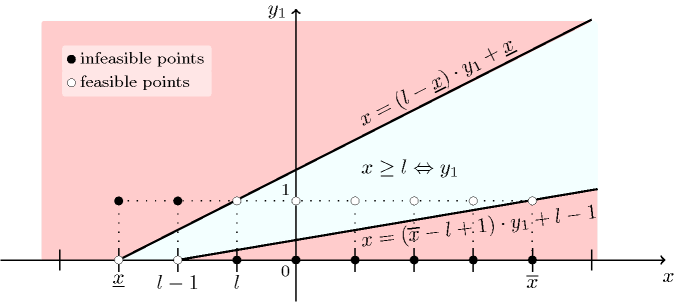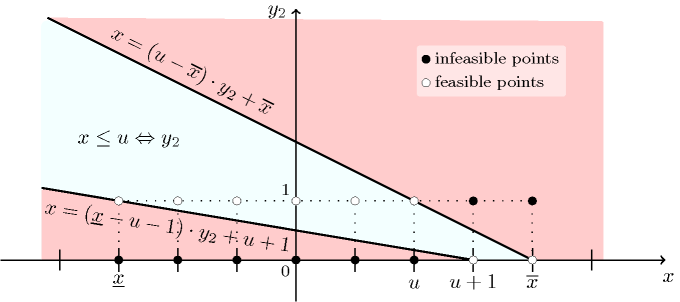## 5.179. in_interval_reified

Origin

Reified version of $\mathrm{𝚒𝚗}_\mathrm{𝚒𝚗𝚝𝚎𝚛𝚟𝚊𝚕}$.

Constraint

$\mathrm{𝚒𝚗}_\mathrm{𝚒𝚗𝚝𝚎𝚛𝚟𝚊𝚕}_\mathrm{𝚛𝚎𝚒𝚏𝚒𝚎𝚍}\left(\mathrm{𝚅𝙰𝚁},\mathrm{𝙻𝙾𝚆},\mathrm{𝚄𝙿},𝙱\right)$

Synonyms

$\mathrm{𝚍𝚘𝚖}_\mathrm{𝚛𝚎𝚒𝚏𝚒𝚎𝚍}$, $\mathrm{𝚒𝚗}_\mathrm{𝚛𝚎𝚒𝚏𝚒𝚎𝚍}$.

Arguments
 $\mathrm{𝚅𝙰𝚁}$ $\mathrm{𝚍𝚟𝚊𝚛}$ $\mathrm{𝙻𝙾𝚆}$ $\mathrm{𝚒𝚗𝚝}$ $\mathrm{𝚄𝙿}$ $\mathrm{𝚒𝚗𝚝}$ $𝙱$ $\mathrm{𝚍𝚟𝚊𝚛}$
Restrictions
 $\mathrm{𝙻𝙾𝚆}\le \mathrm{𝚄𝙿}$ $𝙱\ge 0$ $𝙱\le 1$
Purpose

Enforce the following equivalence, $\mathrm{𝚅𝙰𝚁}\in \left[\mathrm{𝙻𝙾𝚆},\mathrm{𝚄𝙿}\right]⇔𝙱$.

Example
$\left(3,2,5,1\right)$

The $\mathrm{𝚒𝚗}_\mathrm{𝚒𝚗𝚝𝚎𝚛𝚟𝚊𝚕}_\mathrm{𝚛𝚎𝚒𝚏𝚒𝚎𝚍}$ constraint holds since:

• Its first argument $\mathrm{𝚅𝙰𝚁}=3$ is greater than or equal to its second argument $\mathrm{𝙻𝙾𝚆}=2$ and less than or equal to its third argument $\mathrm{𝚄𝙿}=5$ (i.e., $3\in \left[2,5\right]$).

• The corresponding Boolean variable $𝙱$ is set to 1 since condition $3\in \left[2,5\right]$ holds.

Typical
 $\mathrm{𝚅𝙰𝚁}\ne \mathrm{𝙻𝙾𝚆}$ $\mathrm{𝚅𝙰𝚁}\ne \mathrm{𝚄𝙿}$ $\mathrm{𝙻𝙾𝚆}<\mathrm{𝚄𝙿}$
Symmetries
• An occurrence of a value of $\mathrm{𝚅𝙰𝚁}$ that belongs to $\left[\mathrm{𝙻𝙾𝚆},\mathrm{𝚄𝙿}\right]$ (resp. does not belong to $\left[\mathrm{𝙻𝙾𝚆},\mathrm{𝚄𝙿}\right]$) can be replaced by any other value in $\left[\mathrm{𝙻𝙾𝚆},\mathrm{𝚄𝙿}\right]$) (resp. not in $\left[\mathrm{𝙻𝙾𝚆},\mathrm{𝚄𝙿}\right]$).

• One and the same constant can be added to $\mathrm{𝚅𝙰𝚁}$, $\mathrm{𝙻𝙾𝚆}$ and $\mathrm{𝚄𝙿}$.

Reformulation

The $\mathrm{𝚒𝚗}_\mathrm{𝚒𝚗𝚝𝚎𝚛𝚟𝚊𝚕}_\mathrm{𝚛𝚎𝚒𝚏𝚒𝚎𝚍}$ constraint can be reformulated in terms of linear constraints. For convenience, we rename $\mathrm{𝚅𝙰𝚁}$ to $x$, $\mathrm{𝙻𝙾𝚆}$ to $l$, $\mathrm{𝚄𝙿}$ to $u$, and $𝙱$ to $y$. The constraint is decomposed into the following conjunction of constraints:

$\begin{array}{cc}& x\ge l⇔{y}_{1},\hfill \\ & x\le u⇔{y}_{2},\hfill \\ & {y}_{1}\wedge {y}_{2}⇔y.\hfill \end{array}$

We show how to encode these constraints with linear inequalities. The first constraint, i.e., $x\ge l⇔{y}_{1}$ is encoded by posting one of the following three constraints:

$\left\{\begin{array}{ccc}\mathrm{a}\right)\hfill & \hfill \mathrm{if}\underline{x}\ge l:& {y}_{1}=1,\hfill \\ \mathrm{b}\right)\hfill & \hfill \mathrm{if}\overline{x}

On the one hand, cases a) and b) correspond to situations where one can fix ${y}_{1}$, no matter what value will be assigned to $x$. On the other hand, in case c), ${y}_{1}$ can take both values 0 or 1 depending on the value assigned to $x$. As shown by Figure 5.179.1, all possible solutions for the pair of variables $\left(x,{y}_{1}\right)$ satisfy the following two linear inequalities $x\ge \left(l-\underline{x}\right)·{y}_{1}+\underline{x}$ and $x\le \left(\overline{x}-l+1\right)·{y}_{1}+l-1$. The first inequality discards all points that are above the line that goes through the two extreme solution points $\left(\underline{x},0\right)$ and $\left(l,1\right)$, while the second one removes all points that are below the line that goes through the two extreme solution points $\left(l-1,0\right)$ and $\left(\overline{x},1\right)$.

##### Figure 5.179.1. Illustration of the reformulation of the reified constraint $x\ge l⇔{y}_{1}$ with two linear inequalitiesThe second constraint, i.e., $x\le u⇔{y}_{2}$ is encoded by posting one of the following three constraints:

$\left\{\begin{array}{ccc}\mathrm{d}\right)\hfill & \hfill \mathrm{if}\overline{x}\le u:& {y}_{2}=1,\hfill \\ \mathrm{e}\right)\hfill & \hfill \mathrm{if}\underline{x}>u:& {y}_{2}=0,\hfill \\ \mathrm{f}\right)\hfill & \hfill \mathrm{otherwise}:& x\le \left(u-\overline{x}\right)·{y}_{2}+\overline{x}\wedge x\ge \left(\underline{x}-u-1\right)·{y}_{2}+u+1.\hfill \end{array}\right\$

On the one hand, cases d) and e) correspond to situations where one can fix ${y}_{2}$, no matter what value will be assigned to $x$. On the other hand, in case f), ${y}_{2}$ can take both value 0 or 1 depending on the value assigned to $x$. As shown by Figure 5.179.2, all possible solutions for the pair of variables $\left(x,{y}_{2}\right)$ satisfy the following two linear inequalities $x\le \left(u-\overline{x}\right)·{y}_{2}+\overline{x}$ and $x\ge \left(\underline{x}-u-1\right)·{y}_{2}+u+1$. The first inequality discards all points that are above the line that goes through the two extreme solution points $\left(\overline{x},0\right)$ and $\left(u,1\right)$, while the second one removes all points that are below the line that goes through the two extreme solution points $\left(u+1,0\right)$ and $\left(\underline{x},1\right)$.

##### Figure 5.179.2. Illustration of the reformulation of the reified constraint $x\le u⇔{y}_{2}$ with two linear inequalitiesThe third constraint, i.e., ${y}_{1}\wedge {y}_{2}⇔y$ is encoded as:

$\left\{\begin{array}{cc}\mathrm{g}\right)\hfill & y\ge {y}_{1}+{y}_{2}-1,\hfill \\ \mathrm{h}\right)\hfill & y\le {y}_{1},\hfill \\ \mathrm{i}\right)\hfill & y\le {y}_{2}.\hfill \end{array}\right\$

Case g) handles the implication ${y}_{1}\wedge {y}_{2}⇒y$, while cases h) and i) take care of the other side $y⇒{y}_{1}\wedge {y}_{2}$.

uses in its reformulation: $\mathrm{𝚊𝚕𝚕𝚍𝚒𝚏𝚏𝚎𝚛𝚎𝚗𝚝}$ (bound consistency preserving reformulation).